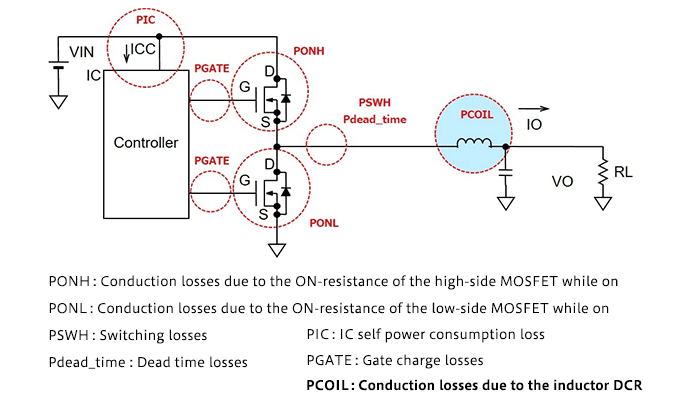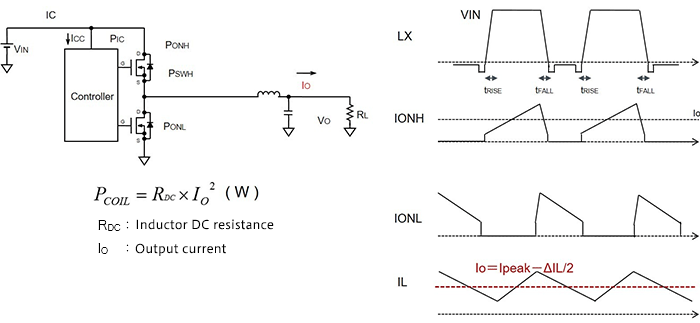Technical Information Site of Power Supply Design

2018.12.20 DC/DC

# Conduction Losses due to the Inductor DCR

Examination of Losses

In this article, we consider losses related to the DCR of output inductors, which are the last of the individual places in a circuit at which losses occur. The output inductor corresponds to the blue area marked "PCOIL" in the diagram below.Conduction Losses due to the Inductor DCR

The inductor DCR, the DC resistance, is the resistance component of the coil. Hence a loss occurs simply because of the DCR and the current flowing in the inductor. The place where the loss occurs is also simply the inductor itself. The flowing current is basically the output current IO. IO can also be determined from the inductor current IL.Of course, the calculation is based on Ohm's law.

In the next article, we will consider overall losses.

#### Key Points:

・Conduction losses occur due to the inductor DCR (DC resistance) and the output current.